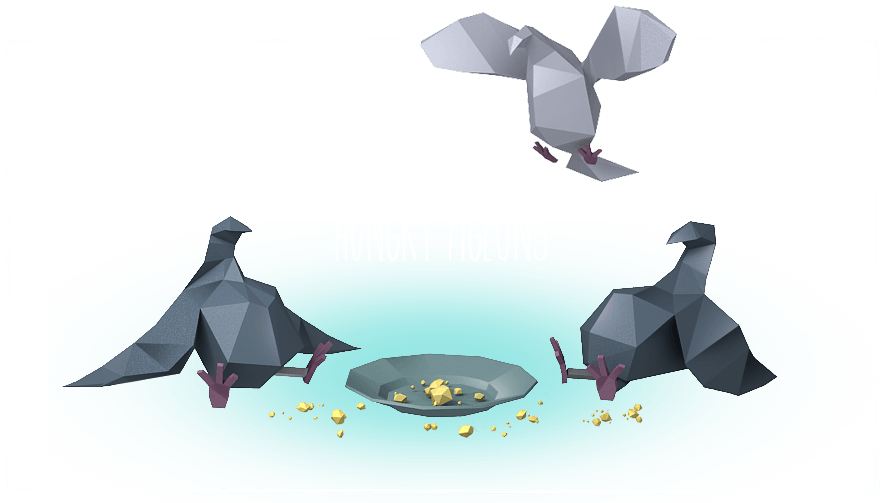• # "Feed Pigeons" ReviewHi, CiO Friends!

On our landing page you can find one mission text with solutions. And this is "Feed Pigeons". I'm not sure why but this mission can trouble new players.

## Description

I start to feed one of the pigeons. A minute later two more fly by and a minute after that another 3. Then 4, and so on (Ex: 1+2+3+4+...). One portion of food lasts a pigeon for a minute, but in case there's not enough food for all the birds, the pigeons who arrived first ate first. Pigeons are hungry animals and eat without knowing when to stop. If I have N portions of bird feed, how many pigeons will be fed with at least one portion of wheat?

## Simple

The simplest way to solve it is using step-by-step model and repeat all stages of feeding. Create minute counter and for each minute increasing we reduce wheat portions and add new pigeons. But we should be careful for the step when we have portions less than pigeons, because for this case we feed "old" pigeons first, even if they are fed already. In our solution "minute" is the same as a quantity of new arrived pigeons.

``````def checkio(portions):
"""Simple and straight"""
fed = minute = pigeons = 0
while portions >= 0:
portions -= pigeons
minute += 1
if portions <= 0:
return fed
if minute < portions:
fed += minute
portions -= minute
else:
fed += portions
return fed
pigeons += minute
return fed
``````

## Sequence

But if we will write solution results for several input values, then we can notice that these numbers create a sequence.

`1, 1, 2, 3, 3, 3, 3, 4, 5, 6, 6, 6, 6, 6, 6, 6, 7, 8, 9, 10, 10, 10, 10, 10, 10, 10, 10, 10...`

I hope you see a pattern. Now we need to write a generator for this and honestly we can receive all answer for 1 to N in one run.

``````def checkio(portions):
step, i, results = 1, 1, []
while len(results) < portions + 1:
results.extend([i] * (i + 1))
results.extend(range(i + 1, i + 1 + step))
step += 1
i += step
return results[portions]
``````

## Math

But this mission can be solved with pure mathematics (ok, almost pure) and O(1) complexity. And @bunnychai proved this with "Arithmetic" solution

``````def checkio(number):
k = int((6 * number) ** (1.0 / 3))
if k * (k + 1) * (k + 2) > 6 * number:
k -= 1
t = k * (k + 1) / 2
s = t * (k + 2) / 3
return t + max(number - s - t, 0)
``````

and an another variant by @DiEvAl in "First" solution. Here you can find an explanation of the math.

``````def checkio(n):
# After m minutes there are m(m+1)/2 birds and we've used m(m+1)(m+2)/6 portions of wheet.
# Solving the equation x(x+1)(x+2)=6n (http://www.wolframalpha.com/input/?i=x%28x%2B1%29%28x%2B2%29%3D6n)
# gives us only 1 positive real root for a > 0:
# x = (sqrt(3) sqrt(243 a^2-1)+27 a)^(1/3)/3^(2/3)+1/(3^(1/3) (sqrt(3) sqrt(243 a^2-1)+27 a)^(1/3))-1
t = ((9*n**2 - 1/27) ** (1/2) + 3*n) ** (1/3)
x = t + 1/(3*t) - 1
m = int(x)
return max(m*(m+1)/2, n - m*(m+1)*(m+2)/6)
``````

## Creative

And as usually "Creative" solutions as dessert. For example this golf solution by @melpon

``````f=lambda n,y=0,i=0:n<y and max(n,y-i+1)or f(n-y,sum(range(i+1)),i+1)
checkio=f
``````

And @veky with his "Optimal" solution, where math twisted with binary search. You can find hints how it's work in comments or ask the author of this solution.

``````def pbs(cond, start=0):
"""last int after start satisfying cond
Precondition: cond(n)=>cond(n-1) & cond(start) & exists n>start not cond(n)
Complexity: max. 2*ld(result-start), calls cond on distinct numbers only"""
step = 1
while cond(start + step): step <<= 1
start += step >> 1
step >>= 2
while step:
if cond(start + step): start += step
step >>= 1
return start
​
def checkio(n):
k = pbs(lambda k: k**3 - k <= 6*n)
return max(n - (k**3 - k)//6, (k**2 - k)//2)
``````

That's all folks. Be careful with Hungry Pigeons ;-)

Valentin Bryukhanov aka Bryukh

Welcome to CheckiO - games for coders where you can improve your codings skills.

The main idea behind these games is to give you the opportunity to learn by exchanging experience with the rest of the community. Every day we are trying to find interesting solutions for you to help you become a better coder.